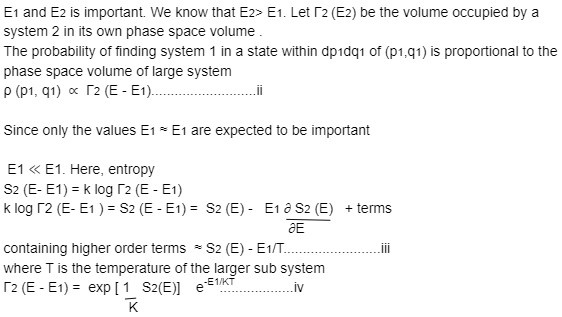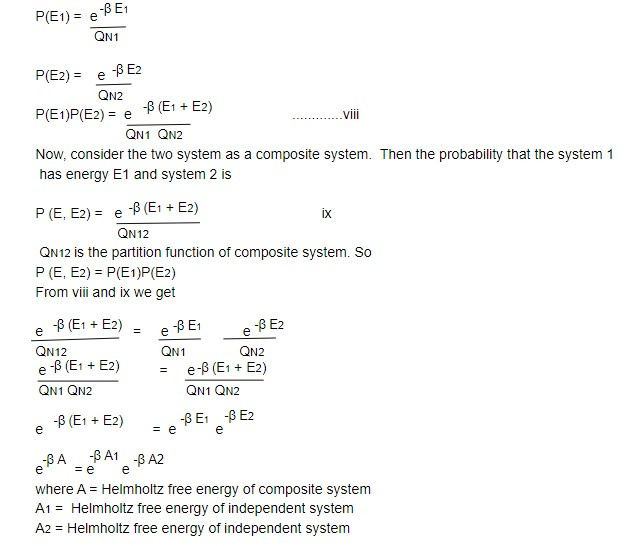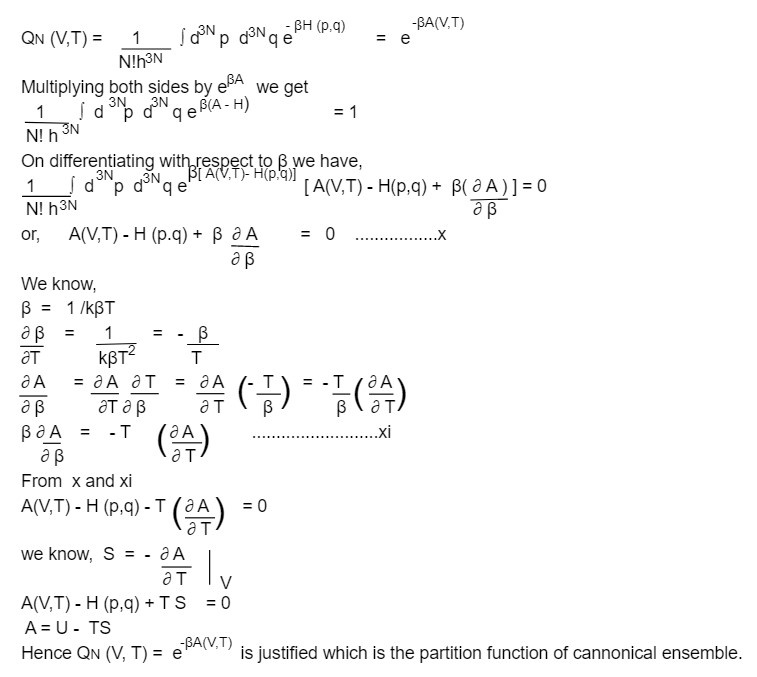# Cannonical Ensemble

The microcannonical ensemble is a general statistical tool but it is often very difficult to use practice because of difficulty in evaluating the volume of phase space. Hence cannonical ensemble is invented by Gibb's which gives the description of a system which is not in isolation but in thermal equilibrium with a larger system.

Let us consider an isolated composite system made up of two sub systems whose Hamiltonians are respectively H1(p1,q1) and H2(p2,q2) with number of particles N1 and N2 respectively. We assume that N2>>N1 but both N1 and N2 are microscopically large.

Consider microcanonical ensemble of the composite system with total energy E and E+ △E. The energy E1 and E2 of the sub system accordingly can have any values satisfying E< (E1 + E2)< E+ △E .......(i)

Although this includes a range of values E1 and E2. We know that one set of value namelyThe first factor is independent of E1 and is thus a constant as far as the small sub system is concerned.

From ii and iv and placing E1 = H1(p,q) we may write

density ρ(p,q) = e -H(p,q)/KT ...................v

The ensemble defined by v whose temperature is determined through contact with hot reservoir is called cannonical ensemble.

The volume in phase space occupied by the cannonical ensemble is called the partition function i.e

where A(V,T) = Helmholtz free energy

Justification of vii rests on the proof of the following points.

1. A is an extensive property

2. A is related to the internal energy U and entropy S = - (∂A/ ∂T)v by the thermodynamics relation

A = U- TS

Justification of 1

Proof: Let us consider two independent system 1 and 2 having number of particles N1 and N2 and partition function QN1 and QN2. Both the system are in thermal equilibrium with the hot reservoir at temperature T.

If P(E1) is the probability that the system 1 has energy E1 and P(E2) is the probability that the system 2 has energy E2. We know,Justification of 2

We have the partition function,This note is taken from Classical Mechanics, MSC physics, Nepal.

This note is a part of the Physics Repository.